# Percentages + volume - math problems

#### Number of problems found: 97

• Feed to hay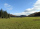How many tons of water do we have to dehydrate from 8 tons of feed, if the original moisture is 54 percent, to get hay with a moisture content of 15 percent? Round to two decimal places.
• The golfThe golf ball with a diameter of 43 mm is packed in a cube-shaped box. What percentage of the box volume is made up of a ball?
• FertilizerI have 4 liters of 10% fertilizer. How much water do I need to add to have a 2% solution?
• PharmacistThe pharmacist had a 12 l vessel herbal lotion, which contained a 30% active ingredient. Her assistant poured 18 liters of herbal lotion containing 45% of the active ingredient into a container. What percentage of herbal lotion was produced?
• Alcohol solutionsWe have to produce 2 liters of 60% alcohol from 55% and 80%. How many of which ones will we use in the solution?
• How many 9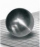How many percent volume remain of a sphere if diameter decrease 3×?
• Iglu - cone tentThe cone-shaped tent is 3 m high, the diameter of its base is 3.2 m. a) The tent is made of two layers of material. How many m2 of fabric is needed for production (including flooring) if 20% needs to be added to the minimum amount due to cutting waste? b)
• Fresh juiceThe seller offers fresh-squeezed juice, which the customer either pours into his own containers or sells in liter plastic bottles, which the customer buys from him. A liter of juice costs 40 CZK more than a bottle. There is no charge for turning the juice
• Exponential decayA tank contains 55 liters of water. Water is flowing out at the rate of 7% per minute. How long does it take to drain the tank?
• The water barrel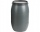The water barrel weighs 122 kg. If we pour 75% of the water out of it, it will weigh 35 kg. What is the weight of the barrel?
• Solutions, mixturesWe have 2 liters of 20% solution available. How much 70% solution do we need to add to it to get a 30% solution?
• Beer tapping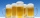When checking compliance with the beer tapping, it was found that 60% of the offered beers were underfilled. The others were fine. Thus, instead of 0.5 l, the volume was 4.4 dcl on average. What was the volume of one average underfilled beer?
• Cylinder in cube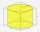Into a paper box in the shape of a cube with an edge of 10 cm is placed a can in the shape of a cylinder with a height of 10 cm and touching all the walls of the cube. What % of the volume of the cube can take up?
• Cube in sphereThe cube is inscribed in a sphere with a radius r = 6 cm. What percentage is the volume of the cube from the volume of the ball?
• Water tanksA different amount of water is poured into each of the three identical vessels. In the first vessel, water fills 30% of its volume and in the second vessel 40% of its volume. The third container contains 19 liters of water. If we distributed the water fro
• Triangular prismThe base of the perpendicular triangular prism is a rectangular triangle with a hypotenuse of 10 cm and one leg of 8 cm. The prism height is 75% of the perimeter of the base. Calculate the volume and surface of the prism.
• Volume of woodEvery year, at the same time, an increase in the volume of wood in the forest is measured. The increase is regularly p% compared to the previous year. If in 10 years the volume of wood has increased by 10%, what is the number p?
• Growth of woodThe annual growth of wood in the forest is estimated at 2%. In how many years will make the forest volume double?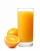Iva added one liter of 100% fruit juice to 3 liters of water. She left two liters of it for Alice and Beata. She added two more liters of water to the remain two liters of lemonade and offered it to other friends. a. What percentage of juice did Alena andThe ball has a radius of 2m. What percentage of the surface and volume is another sphere whose radius is 20% larger?